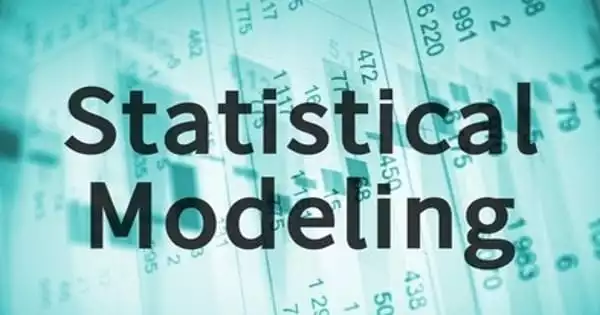# Statistical ModelingRegression analysis is a set of statistical processes used in statistical modeling to estimate the relationships between a dependent variable and one or more independent variables. It is the application of statistical analysis to a dataset. Linear regression is the most common type of regression analysis, in which the line (or a more complex linear combination) that best fits the data according to a specific mathematical criterion is found. When data analysts apply various statistical models to the data they are investigating, they are able to more strategically understand and interpret the information.

Statistical Modeling is the application of statistical analysis to a dataset. It is a mathematical relationship that exists between one or more random variables and other non-random variables.

The use of mathematical models and statistical assumptions to generate sample data and make predictions about the real world is known as statistical modeling. It is a mathematical relationship that exists between one or more random variables and other non-random variables. A statistical model is a set of probability distributions for all possible outcomes of an experiment. The application of statistical modeling to raw data assists data scientists in approaching data analysis strategically by providing intuitive visualizations that aid in identifying relationships between variables and making predictions.

A statistical model is a mathematical representation (or mathematical model) of data that has been observed. The ordinary least squares method, for example, computes the unique line (or hyperplane) that minimizes the sum of squared differences between the true data and that line (or hyperplane). Before developing a statistical model, an analyst must collect or retrieve data from a database, the cloud, social media, or a plain Excel file. Analysts must also understand data structure and management, including how and where data is stored, retrieved, and maintained.

This allows the researcher to estimate the conditional expectation (or population average value) of the dependent variable when the independent variables take on a given set of values for specific mathematical reasons. Less common types of regression employ slightly different procedures to estimate alternative location parameters (e.g., quantile regression or Necessary Condition Analysis) or the conditional expectation across a broader range of non-linear models (e.g., nonparametric regression).

The statistical model can be defined as a collection of results based on aggregated data and population understanding that are used to forecast information in a generalized form. As a result, a statistical model could be an equation or a visual representation of the information based on extensive research conducted over time.

Regression analysis is primarily used for two distinct conceptual purposes. First, regression analysis is widely used for prediction and forecasting, and its application overlaps significantly with the field of machine learning. Second, regression analysis can be used to infer causal relationships between independent and dependent variables in some cases.

Importantly, regressions reveal only relationships between a dependent variable and a set of independent variables in a fixed dataset. A researcher must carefully justify why existing relationships have predictive power for a new context or why a relationship between two variables has a causal interpretation before using regressions for prediction or inferring causal relationships. The latter is especially important when using observational data to estimate causal relationships.